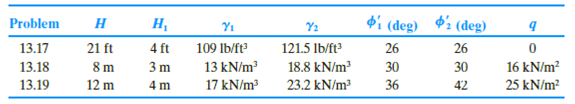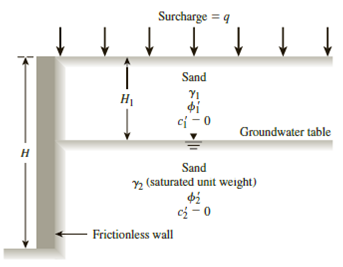Chapter 13, Problem 13.20PPrinciples of Geotechnical Enginee...

9th Edition
Braja M. Das + 1 other
ISBN: 9781305970939

Solutions

Chapter
SectionPrinciples of Geotechnical Enginee...

9th Edition
Braja M. Das + 1 other
ISBN: 9781305970939
Textbook Problem

13.17 through 13.19 A retaining wall is shown in Figure 13.41. For each problem, determine the Rankine active force, Pa, per unit length of the wall and the location of the resultant.Figure 13.4113.20 For the partially submerged backfill in Problem 13.17 (Figure 13.41), determine the Rankine’s passive force per unit length of the wall and the location of the resultant.

To determine

Find the Rankine passive force Pp per unit length of the wall and the location z¯ of the resultant force.

Explanation

Given information:

The height (H) of the retaining wall is 21 ft.

The depth H1 of sand is 4.0 ft.

The unit weight γ1 of the sand is 109lb/ft3.

The sand friction angle ϕ1 is 26°.

The cohesion c1 of sand is 0.

The surcharge pressure (q) is 0.

The depth H2 of saturated sand is 4.0 ft.

The saturated unit weight γ2 of the sand is 121.5lb/ft3.

The saturated sand friction angle ϕ2 is 26°.

The cohesion c2 of saturated sand is 0.

Calculation:

Determine the passive earth pressure coefficient Kp using the formula.

Kp=tan2(45+ϕ2)

Substitute 26° for ϕ.

Ka=tan2(45+26°2)=tan2(45+13)=tan2(58)=2.56

Determine the total stress σo at 0 ft depth using the relation.

σo=0

Determine the pore water pressure at 0 ft depth using the relation.

u=γw×h

Here, γw is the unit weight of the water.

Take the unit weight of the water as 62.4lb/ft3.

Substitute 62.4lb/ft3 for γw and 0 ft for h.

u=62.4×0=0

Determine the effective active earth pressure σa at 0 ft depth using the relation.

σa=σoKa

Substitute 0 for σo and 2.56 for Ka.

σa=0(2.56)=0

Determine the total stress σo at 4 ft depth using the relation.

σo=γ1×H1

Substitute 109lb/ft3 γ1 and 4.0 ft for H1.

σo=109×4.0=436lb/ft2

Determine the pore water pressure at 4 ft depth using the relation.

u=γw×h

Here, γw is the unit weight of the water.

Take the unit weight of the water as 62.4lb/ft3.

Substitute 62.4lb/ft3 for γw and 0 ft for h.

u=62.4×0=0

Determine the effective active earth pressure σa at 4 ft depth using the relation.

σa=σoKa

Substitute 436lb/ft2 for σo and 2.56 for Ka.

σa=436(2.56)=1,116.16lb/ft2

Determine the total stress σo at 21 ft depth using the relation.

σo=γ1×H1+(γ2γw)×H2

Substitute 109lb/ft3 γ1, 4.0 ft for H1, 121.5lb/ft3 γ2, 62.4lb/ft3 for γw, and 17 ft for H2.

σo=109×4.0+(121.562.4)×17=1,440.7lb/ft2

Determine the pore water pressure at 0 ft depth using the relation.

u=γw×h

Substitute 62.4lb/ft3 for γw and 17 ft for h.

u=62.4×17=1,060.8lb/ft2

Determine the effective active earth pressure σa at 24 ft depth using the relation

Still sussing out bartleby?

Check out a sample textbook solution.

See a sample solution

The Solution to Your Study Problems

Bartleby provides explanations to thousands of textbook problems written by our experts, many with advanced degrees!

Get Started

What is a Brale penetrator and what hardness scale uses it?

Precision Machining Technology (MindTap Course List)

What is risk management?

Management Of Information Security

What e agile methods? Are they better than traditional methods? Why or why not?

Systems Analysis and Design (Shelly Cashman Series) (MindTap Course List)

List the steps to use your phone as a mobile hot spot.

Enhanced Discovering Computers 2017 (Shelly Cashman Series) (MindTap Course List)

Why is a slight forward torch angle helpful for cutting?

Welding: Principles and Applications (MindTap Course List)